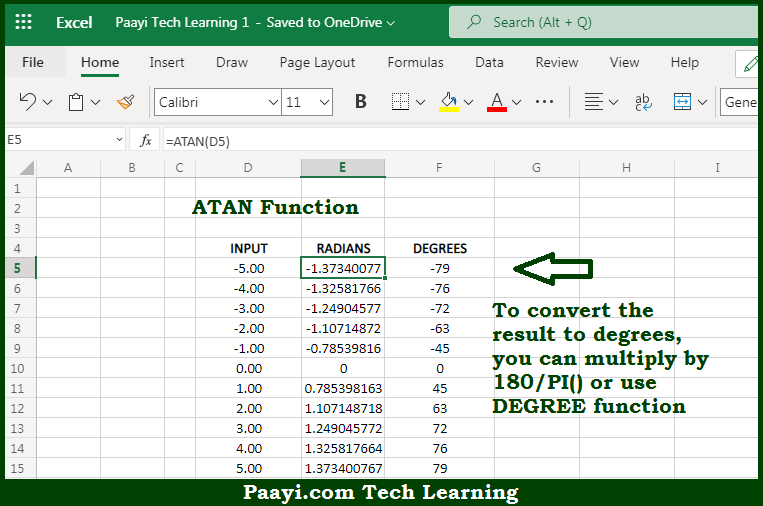# Learn How to Use Microsoft Excel ATAN Function

Written by | 0 Comments | 608 Views

In this article, you will learn how to use the Microsoft Excel ATAN function and its prime function in Microsoft Excel. You will also get to know the Microsoft Excel ATAN function return value and syntax with the help of some examples.

Microsoft Excel ATAN Function

The main purpose of the Microsoft Excel ATAN function is to get the arctangent of a number. That implies, with the help of the ATAN function you can able to return the inverse tangent of the number. It should be noted that the ATAN function is the inverse of the TAN function. In geometry, ATAN returns the angle of a right triangle given the ratio of its opposite side over its adjacent side. So, with the help of the ATAN function, you can able to get inverse TAN values of a number.

Return Value of ATAN Function

The return value will be the angle in radians.

Syntax of ATAN Function

=ATAN(number)

Where the arguments:

• number: This is the number or value of which you want to get the inverse TAN.

## How to Use Microsoft Excel ATAN Function?So we know that Microsoft Excel ATAN function you can able to get the arctangent of a number. That implies, with the help of the ATAN function you can able to return the inverse tangent of the number. It should be noted that the ATAN function is the inverse of the TAN function. In geometry, the function returns the angle of a right triangle given the ratio of its opposite side over its adjacent side. So, with the help of the ATAN function, you can able to get inverse TAN values of a number.